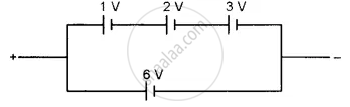# Find the Emf of the Battery Shown in the Figure: - Physics (Theory)

Find the emf of the battery shown in the figure:#### Solution

Net emf of the cells in each row = 6 V
Since the two rows are connected in parallel.
Net emf = emf of each row = 6 V

Concept: Cells, Emf, Internal Resistance
Is there an error in this question or solution?